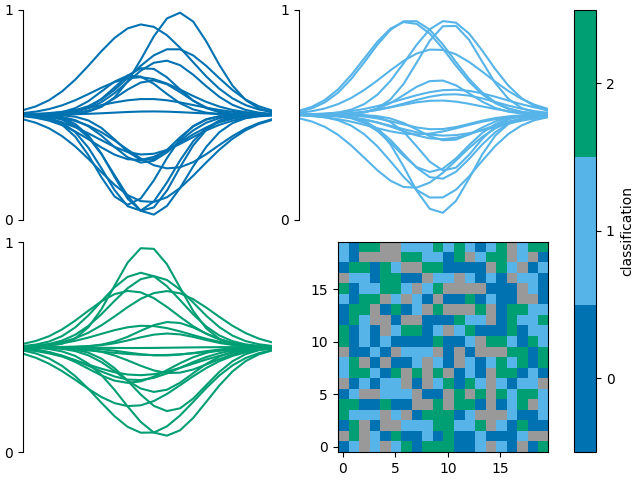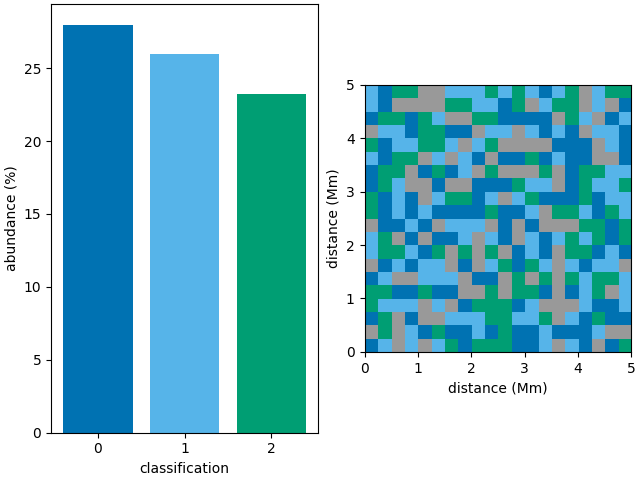Combine multiple classification plots

This is an example showing how to use multiple classification plotting functions in a single figure.

First we shall create a random 3D grid of classifications that can be plotted. Usually you would use a method such as mcalf.models.ModelBase.classify_spectra() to classify an array of spectra.

from mcalf.tests.helpers import class_map as c

t = 1  # One image
x = 20  # 20 coordinates along x-axis
y = 20  # 20 coordinates along y-axis
n = 3  # Possible classifications [0, 1, 2]

class_map = c(t, x, y, n)  # 3D array of classifications (t, y, x)

Next we shall create a random array of spectra each labelled with a random classifications. Usually you would provide your own set of hand labelled spectra taken from spectral imaging observations of the Sun. Or you could provide a set of spectra labelled by the classifier.

from mcalf.tests.visualisation.test_classifications import spectra as s

n = 400  # 200 spectra
w = 20  # 20 wavelength points for each spectrum
low, high = 0, 3  # Possible classifications [0, 1, 2]

# 2D array of spectra (n, w), 1D array of labels (n,)
spectra, labels = s(n, w, low, high)

If a GridSpec returned by the plot_classification function has free space, a new axes can be added to the returned GridSpec. We can then request plot_class_map to plot onto this new axes. The colorbar axes can be set to fig.axes such that the colorbar takes the full height of the figure, as in this case, its colours are the same as the line plots.

import matplotlib.pyplot as plt
from mcalf.visualisation import plot_classifications, plot_class_map

fig = plt.figure(constrained_layout=True)

gs = plot_classifications(spectra, labels, nrows=2, show_labels=False)

plot_class_map(class_map, ax=ax, colorbar_settings={
'ax': fig.axes,
'label': 'classification',
})Out:

<matplotlib.image.AxesImage object at 0x7f90a894d130>

The function mcalf.visualisation.init_class_data`() is intended to be an internal function for generating data that is common to multiple plotting functions. However, it may be used externally if necessary.

from mcalf.visualisation import init_class_data, bar

fig, ax = plt.subplots(1, 2, constrained_layout=True)

data = init_class_data(class_map, resolution=(0.25, 0.25), ax=ax)

bar(data=data, ax=ax)
plot_class_map(data=data, ax=ax, show_colorbar=False)Out:

The following example should be equivalent to the example above,

fig, ax = plt.subplots(1, 2, constrained_layout=True)

bar(class_map, ax=ax)
plot_class_map(class_map, ax=ax, show_colorbar=False,
resolution=(0.25, 0.25))Out:

<matplotlib.image.AxesImage object at 0x7f90ab0f60a0>

Total running time of the script: ( 0 minutes 0.883 seconds)

Gallery generated by Sphinx-Gallery